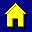3-Space and Spacetime

This short tutorial about the analogy between Euclidean 3 -dimensional space and 4-dimensional spacetime is for those who are familiar with analytical geometry.

Consider two Euclidean coordinate systems, one rotated with respect to the other, as shown in part (a) of the figure. Although the point P has different coordinates, the square of the distance to the origin, x2+y2= x'2+y'2 , is the same in both systems. Also, there are transformations to convert x to x' and y to y' in terms of the angle of rotation.Similarly, in spacetime, velocity is like rotation. The axes, x' and t', of the one frame moving with respect to another are rotated as shown in part (b) of the figure. The rotation is a bit different from Euclidean rotations: the new axes are symmetrical about the dashed line. This line represents the world line of a beam of light originating at the origin.

Like in Euclidean space, there are equations to relate x and t to x' and t', the Lorentz transformations. There is a "distance" in spacetime, called the interval, the square of which is
s2 = x2-c2t2 = x'2-c2t'2.
When s2 is positive, the interval is "spacelike"; when it is negative, the interval is "timelike." This is the same in all (inertial) coordinate systems. So, although the event P has different coordinates, the interval is the same and you can find the coordinates in one frame in terms of the speed and the coordinates in the other frame.

Lines parallel to x, or x' are the lines of simultaneity in the respective coordinate systems. They are shown as dashed lines in part (b) of the figure. This is because they represent the lines where t, or t' is constant. Likewise, the lines parallel to t or t' are lines of fixed location in their respective frames.

Many of the ideas of Euclidean geometry have direct analogies in spacetime geometry. This analogy makes some of the strange ideas of spacetime a little more intuitive.Return to the home page.